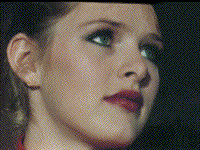This is a child page. You can use Parent in the quick nav bar at the top or the bottom of the page to navigate directly back to the parent of this page. Some child pages are more than one level deep, and in that case will require more than one Parent click to reach the outermost document level.

### § 16.69.3 - Creating Transition Control FilesHere we have a TCF (Transition Control File) that simulates vertical blinds closing. This script is small enough to fit inside of F/x's quick edit window.

Here is how it would be coded:

; v-blinds transition ; Any line that begins with a semicolon are "comments" and ; will not be processed by the transition generator initxy 0.0 0.0 ;you can also put comments on the end of a line repeats 10 1 ;note that parameters are separated by spaces offsets 0.1 0.0 xscales 0.0 1.0 yscales 1.0 1.0 rotate 0 0 line 0.0 0.0 0.0 1.0 line 0.0 1.0 0.1 1.0 line 0.1 1.0 0.1 0.0 line 0.1 0.0 0.0 0.0 ; This code was used, as shown here, to create the animation above

To explain:Key:

You must keep in mind that all measurements are relative values from 0 to 1. 0 being 0% of the width/height of the action image, 1 being 100%.

line 0.0 0.0 1.0 1.0

...would make a line from the top left to the bottom right.

initxy 0.0 0.0
• The first number is the initial X value.
• The second number is the initial Y value
• We begin by initializing X and Y to zero, thus the top corner.
repeats 10 1
• The first number is the number of repeats horizontally
• The second number is the number of repears vertically.
• We set X-repeats to 10 and Y-repeats to 1 so that 10 of the shapes (defined below) will be placed side-by-side
offsets 0.1 0.0
• The first number is the horizontal offset for each repetition
• The second number is the vertical offset for each repetition
• We set x-offset to 0.1 and y-offset to 0.0 so the 10 items will be evenly spaced across the action image.
• y-offset can be zero, as the area occurs only once vertically.
xscales 0.0 1.0 yscales 1.0 1.0
• The first number is the beginning scale.
• The second number is the ending scale.
• Now we have a transition where the 10 evenly spaced areas grow in width with time and have a constant height.
rotate 0 0
• The first number is the beginning angle.
• The second number is the ending angle.
• This transition doesn't rotate but, for example, entering 0 and 360 would cause the transition to make one complete rotation from time 0 to time 1.
line 0.0 0.0 0.0 1.0 line 0.0 1.0 0.1 1.0 line 0.1 1.0 0.1 0.0 line 0.1 0.0 0.0 0.0
• The first number is the starting x.
• The second number is the starting y.
• The third number is the ending x.
• The fourth number is the ending y.
• A line is formed from the starting and ending points, and will constitute an edge of the polygon.
• Together these form a closed rectangle, 1/10th the width and exactly the height of the action image. This polygon, according to the parameters above, will repeat 10 times, spaced evenly across the action image, and grow to its full width over the course of the timeline. All these elements add up to a transition that resembles vertical blinds closing.

More examples